# Chapter 8: Viscous flow in pipes¶

### Example 8.1 Page no.405¶

In :
#given
T1=50.0             #degree farenheit
D=0.73              #in
vol=0.0125        #ft**3
T2=140              #degree farenheit

vis1=2.73*10**-5                          #lb*s/ft**2 at 50 degree farenheit
vis2=0.974*10**-5                        #lb*s/ft**2 at 140 degree farenheit

#calculation
import math
#for 50 degree farenheit
#if flow is laminar, maximum Re=2100 Re=d*V*D/vis
V1=2100*vis1/(1.94*D/12)
t1=vol/(math.pi*((D/12)**2)/4*V1)
#if flow is turbulent, minimum Re=4000
V2=4000*vis1/(1.94*D/12)
t2=vol/(math.pi*((D/12)**2)/4*V2)

#for 140 degree farenheit
#if flow is laminar, maximum Re=2100 Re=d*V*D/vis
V3=2100*vis2/(1.94*D/12)
t3=vol/(math.pi*((D/12)**2)/4*V3)
#if flow is turbulent, minimum Re=4000
V4=4000*vis2/(1.94*D/12)
t4=vol/(math.pi*((D/12)**2)/4*V4)

#result
print("For laminar flow")
print "The time taken to fill the glass at 50 degree F=",round(t1,2),"seconds"
print "The time taken to fill the glass 100 degree F=",round(t3,2),"seconds"
print ("For turbulent flow:")
print "The time taken to fill the glass at 50 degree F=",round(t2,2),"seconds"
print "The time taken to fill the glass at 140 degree F=",round(t4,2),"seconds"

For laminar flow
The time taken to fill the glass at 50 degree F= 8.85 seconds
The time taken to fill the glass 100 degree F= 24.81 seconds
For turbulent flow:
The time taken to fill the glass at 50 degree F= 4.65 seconds
The time taken to fill the glass at 140 degree F= 13.03 seconds


### Example 8.2 Page no.412¶

In :
vis=0.4                    #Ns/(m**2)
d=900                     #kg/(m**3)
D=0.02                   #m
Q=2.0*(10**-5)      #(m**3)/s
x1=0
x2=10                   #m
p1=200                 #kPa
x3=5                     #m

#Calculation
import math
V=Q/(math.pi*(D**2)/4)        #m/s
Re=d*V*D/vis
print "a) Reynolds number =",round(Re,0),"Hence the flow is laminar."

pdiff=128*vis*(x2-x1)*Q/(math.pi*(D**4)*1000)
#for part b0 p1=p2 Q=math.pi*(pdiff-(sw*l*math.sin(ang)))*(D**4)/(128*vis*l)
ang=(math.asin(-128*vis*Q/(math.pi*d*9.81*(D**4))))*180/math.pi
#since sin(ang) doesn= not depend on pdiff, the the pressure is constant all along the pipe
#hence for c)
p3=p1              #kPa

#result
print "The pressure drop required if the pipe is horizontal=",round(pdiff,1),"kpa"
print "b) The angle of the hill the pipe must be on if the oil is to flow at the same rate as a) but with (p1=p2) =",round(ang,2),"degree"
print "c) For conditions of part b), the pressure at x3=5 m = ",round(p3,3),"kpa"

a) Reynolds number = 3.0 Hence the flow is laminar.
The pressure drop required if the pipe is horizontal= 20.4 kpa
b) The angle of the hill the pipe must be on if the oil is to flow at the same rate as a) but with (p1=p2) = -13.34 degree
c) For conditions of part b), the pressure at x3=5 m =  200.0 kpa


### Example 8.3 Page no.416¶

In :
%matplotlib inline

Welcome to pylab, a matplotlib-based Python environment [backend: module://IPython.zmq.pylab.backend_inline].

In :
from numpy import*
T=array([60,80,100,120,140,160])        #degree F
d=array([2.07,2.06,2.05,2.04,2.03,2.02])#(slugs/(ft**3))
vis=array([0.04,0.019,0.0038,0.00044,0.000092,0.000023])#lb*sec/(ft**2)
Q=0.5                            #(ft**3)/sec
T1=100.0                           #degree F
l=6.0                              #ft
D=3.0                              #in

#Calculation
import math
pdiff=128*vis*l*Q/(math.pi*(D/12)**4)
print "The pressure difference is ",round(pdiff,0),"lb/ft**2"
V=Q/(math.pi*((D/12)**2)/4)     #ft/sec
Re=d*V*(D/12)/vis
print"The reynolds number=",round(Re,0),"< 2100 hence the flow is laminar"
stress=pdiff*(D/12)/(4*l)      #lb/(ft**2)
print "The wall stress for the given Q and T =",round(stress,2),"lb/(ft**2)"
Fp=(math.pi/4)*((D/12)**2)*pdiff          #lb
Fv=(2*math.pi)*((D/24))*l*stress        #lb

#result
print "The net pressure force =",round(Fp,2),"lb"
print "The net viscous/shear force =",round(Fv,2),"lb"

#PLot
T=[60,80,100,120,140,160]
K=[0.0004125,0.00086842,0.0043421,0.0375,0.173,0.695]
xlabel("T  F")
ylabel("K  ft**5/lbs")
plt.xlim((60,180))
plt.ylim((0.0001,1))
a=plot(T,K)
show(a)

The pressure difference is  119.0 lb/ft**2
The reynolds number= 1374.0 < 2100 hence the flow is laminar
The wall stress for the given Q and T = 1.24 lb/(ft**2)
The net pressure force = 5.84 lb
The net viscous/shear force = 5.84 lb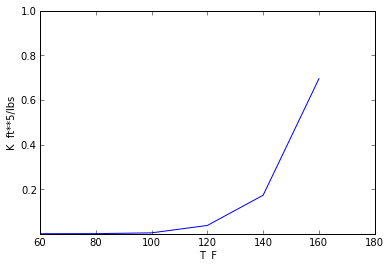### Example 8.4 Page no.427¶

In :
#Given
T=20.0                                       #degree C
d=998.0                                     #kg/(m**3)
kvis=1.004*(10**-6)                   #(m**2)/s where kvis=kinematic viscosity
D=0.1                                        #m
Q=0.04                                      #(m**3)/sec
l=1                                         #m length
#calculation
import math
from scipy import integrate
uf=(stress/d)**0.5                    #m/sec where uf is frictional velocity
ts=5*kvis*1000/(uf)                   #mm where ts is the thickness of the viscous sublayer
print "The thickness of the viscous sublayer=",round(ts,2),"mm"
V=Q/(math.pi*(D**2)/4)               #m/s
Re=V*D/kvis
print "The reynolds number=",round(Re,0),"hence the flow is turbulent."

n=8.4                                #from turbulent flow velocity profile diagram
#Q=(math.pi)*(R**2)*V
R=1                                  #say
def f1(r):
return(1/R**2*(((1-r/R)**(1/n))*2*math.pi*r))
q_=q
x=round(q_,2)/math.pi                          #x=V/Vc
Vc=V/x
print "The approximate centerline velocity=",round(Vc,2),"m/s"

#C
r=0.025                                     #m
stress1=(2*stress*r)/D               #N/(m**2)
#d(uavg)/dr=urate=-(Vc/(n*R))*((1-(r/R))**((1-n)/n)) where uavg=average velocity
urate=-(Vc/(n*(D/2)))*((1-(r/(D/2)))**((1-n)/n))           #s**(-1)
stresslam=-(kvis*d*urate)                                          #N/(m**2)
stressratio=(stress1-stresslam)/stresslam

#result
print "The ratio of teh turbulent to laminar stress at a point\nmidway between the centreline and the pipe wall =",round(stressratio,0)

The thickness of the viscous sublayer= 0.02 mm
The reynolds number= 507267.0 hence the flow is turbulent.
The approximate centerline velocity= 6.04 m/s
The ratio of teh turbulent to laminar stress at a point
midway between the centreline and the pipe wall = 1219.0


### Example 8.5 Page no.435¶

In :
D=4.0                         #mm
V=50.0                      #m/sec
l=0.1                      #m
d=1.23                   #kg/(m**3)
vis=1.79/100000    #N*sec/(m**2)
Re=d*V*(D*10**-3)/vis
#if flow is laminar
f=64/Re
pdiff=f*l*0.5*d*(V**2)/((D*10**-3)*1000)        #kPa

#result
print "The pressure drop if the flow is laminar=",round(pdiff,3),"kpa"

#if flow is turbulent
#roughness=0.0015 hence f=0.028
f1=0.028
pdiff1=f1*l*0.5*d*(V**2)/((D/1000)*1000)   #kPa
print "The pressure drop if flow is turbulent=",round(pdiff1,3),"kpa"

The pressure drop if the flow is laminar= 0.179 kpa
The pressure drop if flow is turbulent= 1.076 kpa


### Example 8.6 Page no.446¶

In :
A=[22,28,35,35,4,4,10,18,22]
V=[36.4,28.6,22.9,22.9,200,200,80,44.4,36.4]
g=32.2                        #ft/s**2  gravitational constant
SG=0.0765                      #spesific gravity
hl=(0.2*(V**2+V**2+V**2+V**2)+0.6*V**2+0.2*V**2+4*V**2)/(2*g)
pdiff=SG*hl/144                          #psi
Pa=SG*A*V*hl

#result
print "The value of (p1-p9)=",round(pdiff,3),"psi"
print "The horsepower supplied to the fluid by the fan=",round(Pa,1)/550,"hp"

#Plot
V=[50,100,150,200,250,300]
Pa=[0,10,25,62.3,120,220]
a=plot(V,Pa)
xlabel("q ft**3/s")
ylabel("d (ft)")
plt.xlim((0,300))
plt.ylim((0,250))

show(a)

The value of (p1-p9)= 0.297 psi
The horsepower supplied to the fluid by the fan= 62.272 hp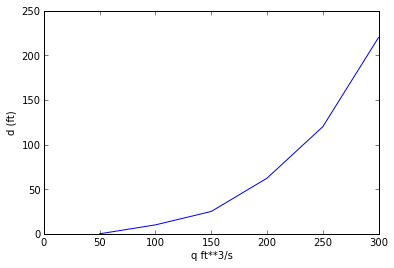### Example 8.7 Page no.449¶

In :
T=120.0                                #degree F
D=8.0                                 #in
vavg=10.0                             #ft/s
roughness=0
kvis=1.89*10**-4                      #(ft**2)/s
Re=vavg*(D/12)/kvis
g=32.2                                #ft/s**2 gravitational constant
#Calculation
import math
#from this value of Re and roughness/D=0, and using Moody's chart
f=0.022
x=f*(vavg**2)/(D*2*32.2/12)            # x=hl/l
#Dh=4*A/P=4*(a**2)/(4*a)=a
y=(math.pi*((D/12)**2)*vavg)/(4)  # y=Vs/a**2
z=x*2*g/y**2                            #z=f/a**5
f=0.023
a=(f/z)**(0.2)

#result
print "The duct size(a) for the square duct if the head loss per foot\n remains the same for the pipe and the duct=",round(a,3),"ft"

The duct size(a) for the square duct if the head loss per foot
remains the same for the pipe and the duct= 0.611 ft


### Example 8.8 Page no.451¶

In :
T=60                          #degree F
D=0.0625                  #ft
Q=0.0267                 #(ft**3)/sec
Df=0.5                     #in
l1=15.0                    #ft
l2=10.0                     #ft
l3=5.0                      #ft
l4=10.0                   #ft
l5=10.0                   #ft
l6=10.0                   #ft

#calculation
import math
V1=Q/(math.pi*(D**2)/4)            #ft/sec
V2=Q/(math.pi*((Df/12)**2)/4)    #ft/sec
d=1.94                                        #slugs/ft
vis=2.34*10**-5                         #lb*sec/(ft**2)
Re=d*V1*D/vis
print "The reynolds number =",round(Re,3),"hence the flow is turbulent"

#applying energy equation between points 1 and 2
#when all head losses are excluded
p1=(d*32.2*(l2+l4))+(0.5*d*((V2**2)-(V1**2)))         #lb/(ft**2)
print "a)The pressure at point 1 when all head losses are neglected=",round(p1,3),"lb/ft**2"

#if major losses are included
f=0.0215
hLmajor=f*(l1+l2+l3+l4+l5+l6)*(V1**2)/(D*2*32.2)
p11=p1+(d*32.2*hLmajor)           #lb/(ft**2)

#result
print "b)The pressure at point 1 when only major head losses are included=",round(p11/144,1),"psi"

#if major and minor losses are included
KLelbow=1.5
KLvalve=10
KLfaucet=2
hLminor=(KLvalve+(4*KLelbow)+KLfaucet)*(V1**2)/(2*32.2)
p12=p11+(d*32.2*hLminor)#lb/(ft**2)
print "c)The pressure at point 1 when both major and minor head losses are included=",round(p12/144,1),"psi"
H=(p1/(32.2*1.94))+(V1*V1/(2*32.2))#ft

#Plot
import matplotlib.pyplot as plt

D=[0,15,15,25,25,30,30,40,40,50,50,60]
P=[30.5,27.8,27.1,21.0,20.2,19.3,18.5,12.4,11.7,9.93,4.84,3.09]
xlabel("distance along pipe from point  ft")
ylabel("P  hP")
plt.xlim((-0.5,60))
plt.ylim((0,32))
a=plot(D,P)
plt.text(40,28, '-(a)no losses')
plt.text(40,25, '-(c)including all losses')

plt.text(0,30.5, '30.5')
plt.text(15,27.8, '27.8')
plt.text(15,27.1, '27.1')
plt.text(25,21.0, '21.0')
plt.text(25,20.2, '20.2')
plt.text(30,19.3, '19.3')
plt.text(30,18.5, '18.5')
plt.text(40,12.4, '12.4')
plt.text(40,11.7, '11.7')
plt.text(50,9.93, '9.93')
plt.text(50,4.84, '4.84')
plt.text(60,3.09, '3.09')
plt.text(0,22, 'pressure loss')
plt.grid()

D=[0,15,25,30,40,50,60]
P=[10.7,10.7,6.37,6.37,2.07,2.07,2.07]
xlabel("distance along pipe from point  ft")
ylabel("P  hP")
plt.xlim((-0.5,60))
plt.ylim((0,32))
a=plot(D,P)
plt.text(0,10.7,  '10.7')
plt.text(15,10.7, '10.7')
plt.text(25,6.37, '6.37')
plt.text(30,6.37, '6.37')
plt.text(40,2.07, '2.07')
plt.text(50,2.07, '2.07')
plt.text(60,2.07, '2.07')
plt.text(5,5, 'Elevation and Kinetic Energy')
show(a)
# 2nd plot
import numpy as np
import matplotlib.pyplot as plt

fig = plt.figure()

D1=[0,15,15,25,25,30,30,40,40,50,50,60,60]
P1=[71.6,67,63,60,58,57,55,53,50,45,31,28,26]
xlabel("distance along pipe from point 1 ft")
ylabel("Elevetion to Energy line H ft")
plt.xlim((0,60))
plt.ylim((0,80))
ax.annotate('slope due to pipe friction', xy=(8, 70), xytext=(15,75),
arrowprops=dict(facecolor='black', shrink=0.000),
)
ax.annotate('sharp drop due to component loss', xy=(15, 65), xytext=(20,65),
arrowprops=dict(facecolor='black', shrink=0.000),
)
ax.annotate('Energy line including all losses', xy=(35, 55), xytext=(40,53),
arrowprops=dict(facecolor='black', shrink=0.000),
)

a1=plot(D1,P1)

D1=[0,60]
P1=[26,26]
xlabel("distance along pipe from point 1 ft")
ylabel("Elevetion to Energy line H ft")
plt.xlim((0,60))
plt.ylim((0,80))
a1=plot(D1,P1)

ax.annotate('Energy line with no losses', xy=(10, 25), xytext=(10,10),
arrowprops=dict(facecolor='black', shrink=0.000),
)

show(a1)

The reynolds number = 45094.88 hence the flow is turbulent
a)The pressure at point 1 when all head losses are neglected= 1547.821 lb/ft**2
b)The pressure at point 1 when only major head losses are included= 21.3 psi
c)The pressure at point 1 when both major and minor head losses are included= 30.5 psi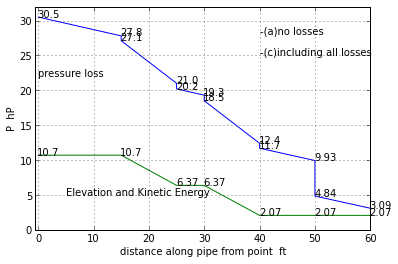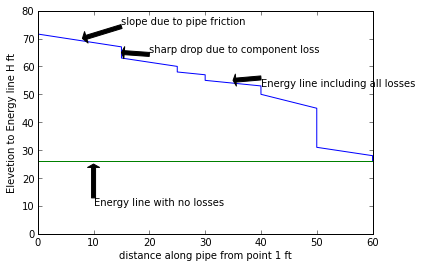### Example 8.9 Page no.453¶

In :
T=140.0                           #degree F
sw=53.7                           #lb/(ft**3)
vis=8*10**-5                    #lb*sec/(ft**2)
l=799                               #miles
D=4.0                              #ft
Q=117.0                          #(ft**3)/sec
V=9.31                            #ft/sec
#energy equation=> hp=hL=f*(l/D)*((V**2)/(2*g))
f=0.0125
hp=f*(l*5280/D)*((V**2)/(2*32.2))        #ft,  in book ,calculation  mistake
Pa=sw*Q*round(hp,2)/550                                  #hp

#Result
print "The horsepower required to drive the system=",round(Pa,3),"hp"

#Plot
import matplotlib.pyplot as plt
fig = plt.figure()

D=[2,3,4,5,6]
P=[3.2*10**6,0.8*10**6,0.202*10**6,0.15*10**6,0.1*10**6]
xlabel("D  ft")
ylabel("P  hP")
plt.xlim((0,6))
plt.ylim((0,4*10**6))
ax.plot(, [0.202*10**6], 'o')
ax.annotate('(4ft,2.02*10**5 hp)', xy=(4,0.25*10**6))
a=plot(D,P)
show(a)

The horsepower required to drive the system= 202694.35 hp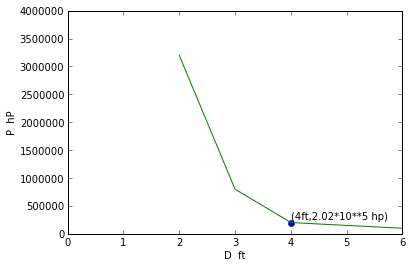### Example 8.10 Page no.454¶

In :
D=4.0                        #in
l=20                           #ft
n=4.0                         #number of 90 degree elbows
h=0.2                        #in
T=100                      #degree F

#calculation
import math
#energy equation between the inside of the dryer and the exit of the vent pipe
p1=(h/12)*62.4         #lb/(ft**2)
KLentrance=0.5
KLelbow=1.5
sw=0.0709              #lb/(ft**3)
f=0.022                  #assumption
#hence,
V=((p1/sw)*2*32.2/(1+(f*l/(D/12))+KLentrance+(n*KLelbow)))**0.5       #ft/sec
Q=V*(math.pi*((D/12)**2)/4)          #(ft**3)/sec

#result
print "The flowrate=",round(Q,2),"ft**3/s"

The flowrate= 0.9 ft**3/s


### Example 8.11 Page no.456¶

In :

D=1.0                      #ft
l=300.0                    #ft
f=0.02                     #moody factor
z1=90.0                   #ft
g=32.2                     #ft/s**2, gravitational constant
sw=62.4                    #lb/ft**3, specific heat
import numpy
#calculation
from scipy.optimize import fsolve
#energy equation between the surface of the lake and the outlet of the pipe
#p1=V1=p2=z2=0  V2=V
a=f*l/(D*2*g)                       #a=hl/V**2
b=Pa*550/(sw*math.pi*(D**2)/4)    #b=ht/V
#from bernouli eq.  f=0.109*V**3-90*V+561
def f(V1):
f=0.109*V1**3-90*V1+561
return(f)
V1=fsolve(f,10)
def f1(V2):
f1=0.109*V2**3-90*V2+561
return(f)
V2=fsolve(f,20)

Q1=(math.pi*(D**2)/4)*V1         #(ft**3)/sec
Q2=(math.pi*(D**2)/4)*V2        #(ft**3)/sec
print "The possible flowrates are=",round(Q1,1),"ft**3/s" "and ",round(Q2,1),"ft**3/s"

The possible flowrates are= 5.166 ft**3/sand  19.537 ft**3/s


### Example 8.12 Page no.457¶

In :
roughness=0.0005                #ft
Q=2.0                            #(ft**3)/sec
pd=0.5*144                      #lb/ft**2  where pd=pressure drop
l=100                           #ft
d=0.00238                      #slugs/(ft**3)
vis=3.74*(10**(-7))            #lb*sec/(ft**2)

#calculation
x=Q/(math.pi/4)                #where x =V*(D**2)
#energy equation with z1=z2 and V1=V2
D=0.404*f**(1/5)
#Calculation
y=(l*d*(x**2)*0.5/(pd))       #where y=(D**5)/f
f=0.027                        #using reynolds number, roughness and moody's chart
D=((l*d*(x**2)*0.5/(pd))*f)**(0.2)

#Result
print "The diameter of the pipe should be =",round(D,3),"ft"

#Plot
q=[0.1,0.5,2,3]
d=[0.06,0.09,0.196,0.225]
a=plot(q,d)
xlabel("q ft**3/s")
ylabel("d (ft)")
plt.xlim((0,3))
plt.ylim((0,0.25))

show(a)

The diameter of the pipe should be = 0.196 ft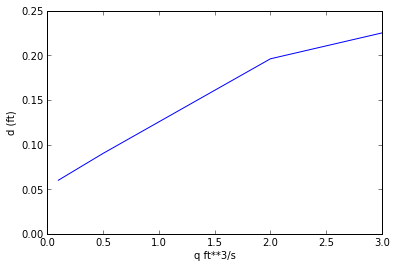### Example 8.13 Page no.458¶

In :
T=60.0                       #degree F
kvis=1.28*(10**(-5))         #(ft**2)/sec
l=1700.0                     #ft
roughness=0.0005             #ft
Q=26.0                       #(ft**3)/sec
n=4.0                        #number of flanged 45 degree elbows
z1=44.0                      #ft

#Calculation
#V=31.1/D*2                   #where V=Q/A
#f=0.0052*D**5-0.00135*D      #eleminating V

#calculation
#assume f=0.052               #(moody chart) hit and trial method
from scipy.optimize import fsolve
def f(D):
f=0.0052*D**5-0.00135*D-0.052
return(f)
D=fsolve(f,1)

#result
print "Pipe Diameter is ",round(D,1),"ft"

#Plot
l=[300,1000,1700,2000]
d=[1.2,1.45,1.63,1.72]
a=plot(l,d)
xlabel("l ft")
ylabel("d (ft)")
plt.xlim((0,2000))
plt.ylim((0,1.8))
show(a)

DPipe Diameter is  1.6 m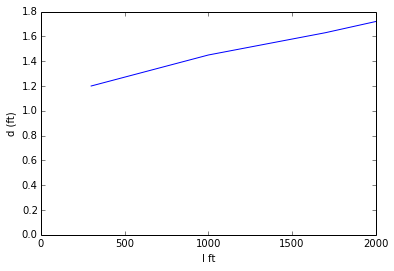### Example 8.14 Page no.462¶

In :
D=1              #ft
f=0.02
z1=100           #ft
z2=20            #ft
z3=0             #ft
l1=1000          #ft
l2=500           #ft
l3=400           #ft

#Calculation
from scipy.optimize import fsolve
import math
#V1+V2=V3                      #from eq of continuity ,because diameter are same
#V1**2+0.4*V3**2=322          #from energy eq..........(1)
#0.5*V2**2+0.4*V3**2=64.4     for fluid flowing B to c.........(2)
# V1**2+0.5*V2**2=258          #...............(3)
#From b and  bernouli eq.  f=V**4-460*V**2+3748
def f(V2):
f1=V2**4-460*V2**2+3748
return(f1)
V2=fsolve(f,2)
V1=math.sqrt(258-0.5*V2**2)       #from eq 3
A=(math.pi/4*(D**2))              #  ft**2, area
Q1=V1*A
Q2=V2*A
Q3=Q1-Q2
print "Flow out of A reservoir =",round(Q1,1), "(ft**3)/sec"
print "Flow  into B reservoir =",round(Q2,2), "(ft**3)/sec"
print "Flow  into C reservoir =" ,round(Q3,1),"(ft**3)/sec"

Flow out of A reservoir = 12.5 (ft**3)/sec
Flow  into B reservoir = 2.26 (ft**3)/sec
Flow  into C reservoir = 10.3 (ft**3)/sec


### Example 8.15 Page no.467¶

In :
D=60.0                 #mm
pdiff=4                  #kPa
Q=0.003               #(m**3)/sec
d=789                 #kg/(m**3)

#calculation
import math
vis=1.19*(10**(-3))   #N*sec/(m**2)
Re=d*4*Q/(math.pi*D*vis)
#assuming B=dia/D=0.577, where dia=diameter of nozzle, and obtaining Cn from Re as 0.972
Cn=0.972
B=0.577
dia=((4*Q/(Cn*math.pi))/((2*pdiff*1000/(d*(1-(B**4))))**0.5))**0.5

#result
print "Diameter of the nozzle=",round(dia*1000,1),"mm"

Diameter of the nozzle= 34.1 mm